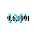### leetcode Question 101: Spiral Matrix II

Spiral Matrix II

Given an integer n, generate a square matrix filled with elements from 1 to n2 in spiral order.
For example,
Given n = 3,
You should return the following matrix:
[
[ 1, 2, 3 ],
[ 8, 9, 4 ],
[ 7, 6, 5 ]
]

Analysis:
Having solved the previous problem. This problem seems easier than the last one.

The key idea is to consider the single step ----- "Which direction to go filling the next number?"
There are only four directions.
Be careful with one thing----- you keep going one direction until meet the end.

Code(Updated 2013.10):
class Solution {
public:
vector<vector<int> > generateMatrix(int n) {
// IMPORTANT: Please reset any member data you declared, as
// the same Solution instance will be reused for each test case.
vector<vector<int> > res(n,vector<int>(n,0));
if (n==0){return res;}
int i=1;
int x = 0;
int y = 0;
res=i++;
while (i<=n*n){
while (y+1<n && res[x][y+1]==0){   // keep going right
res[x][++y]=i++;
}
while (x+1<n && res[x+1][y]==0){   // keep going down
res[++x][y]=i++;
}
while (y-1>=0 && res[x][y-1]==0){  // keep going left
res[x][--y]=i++;
}
while (x-1>=0 && res[x-1][y]==0){  // keep going up
res[--x][y]=i++;
}
}
return res;
}
};


1.2.3.